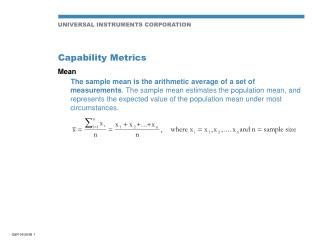DownloadDownload PresentationCapability Metrics

# Capability Metrics

Télécharger la présentation## Capability Metrics

- - - - - - - - - - - - - - - - - - - - - - - - - - - E N D - - - - - - - - - - - - - - - - - - - - - - - - - - -
##### Presentation Transcript

1. Capability Metrics • Mean • The sample mean is the arithmetic average of a set of measurements. The sample mean estimates the population mean, and represents the expected value of the population mean under most circumstances.

2. Capability Metrics (Continued) • Accuracy • A measure of the difference between the mean of the process and the target value. Typically, the target value is centered between the upper and lower specifications. (Note: for some customers, accuracy has a different meaning.)

3. Capability Metrics (Continued) • Standard Deviation • A measure of the spread or dispersion of the values of a random variable. It is defined on a sample by the equation given here. Alternatively, the standard deviation is the expected distance any one observation falls from the mean. • Repeatability • Repeatability is the ability to repeat the same placement by the same process, at or near the same time. It is usually measured by the standard deviation of the process. (Note: for some customers, it is measured by three standard deviations.)

4. Capability Metrics (Continued) • Cpk • Process capability index, which is a measure of the process’ ability to produce product within specification. If the process distribution is known, then a ppm defective rate can be calculated for the process using the Cpk. • where s = the standard deviation of the sample

5. Capability Metrics (Continued) • Cp • The Cp is a capability index which compares the spread of the process to the distance between the upper and lower specifications. If the mean could be shifted to the midpoint between the specification limits (the mean could be dialed out), then the Cpk value would increase to the value of the Cp. In this way, the Cp is the highest value that the Cpk can take without reducing the process standard deviation. • where s = the standard deviation of the sample

6. Capability Metrics (Continued) • Sigma Level • A process capability index, which is a measure of the process’ ability to produce product within specifications. If the process is known, then a ppm defective rate can be calculated for the process using the sigma level. (Note: Motorola uses a different method for obtaining the ppm defect rate. To avoid confusion, it is better to speak in terms of Cpk.) • where s = the standard deviation of the sample